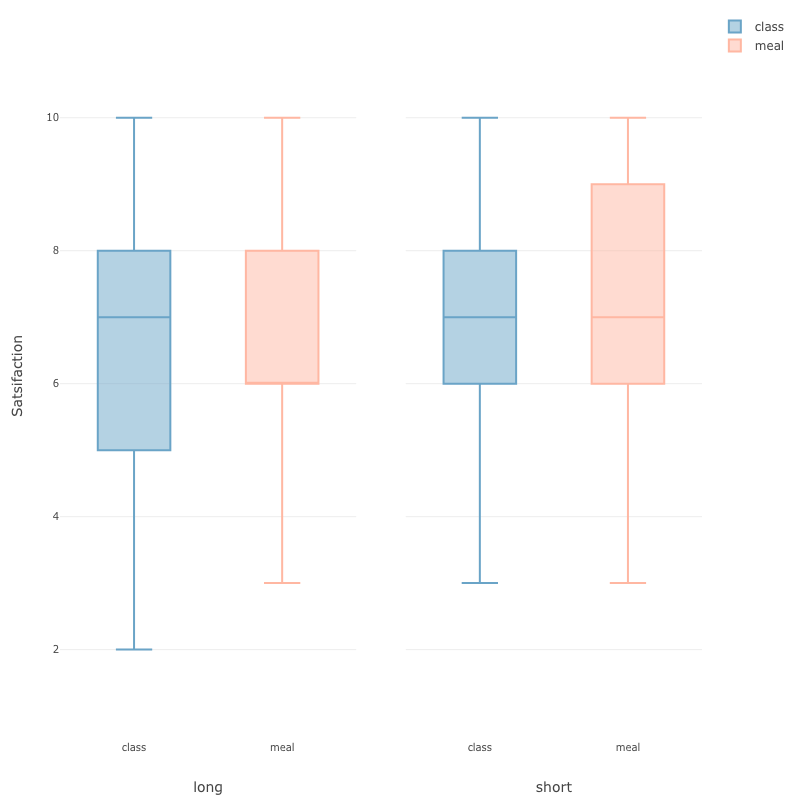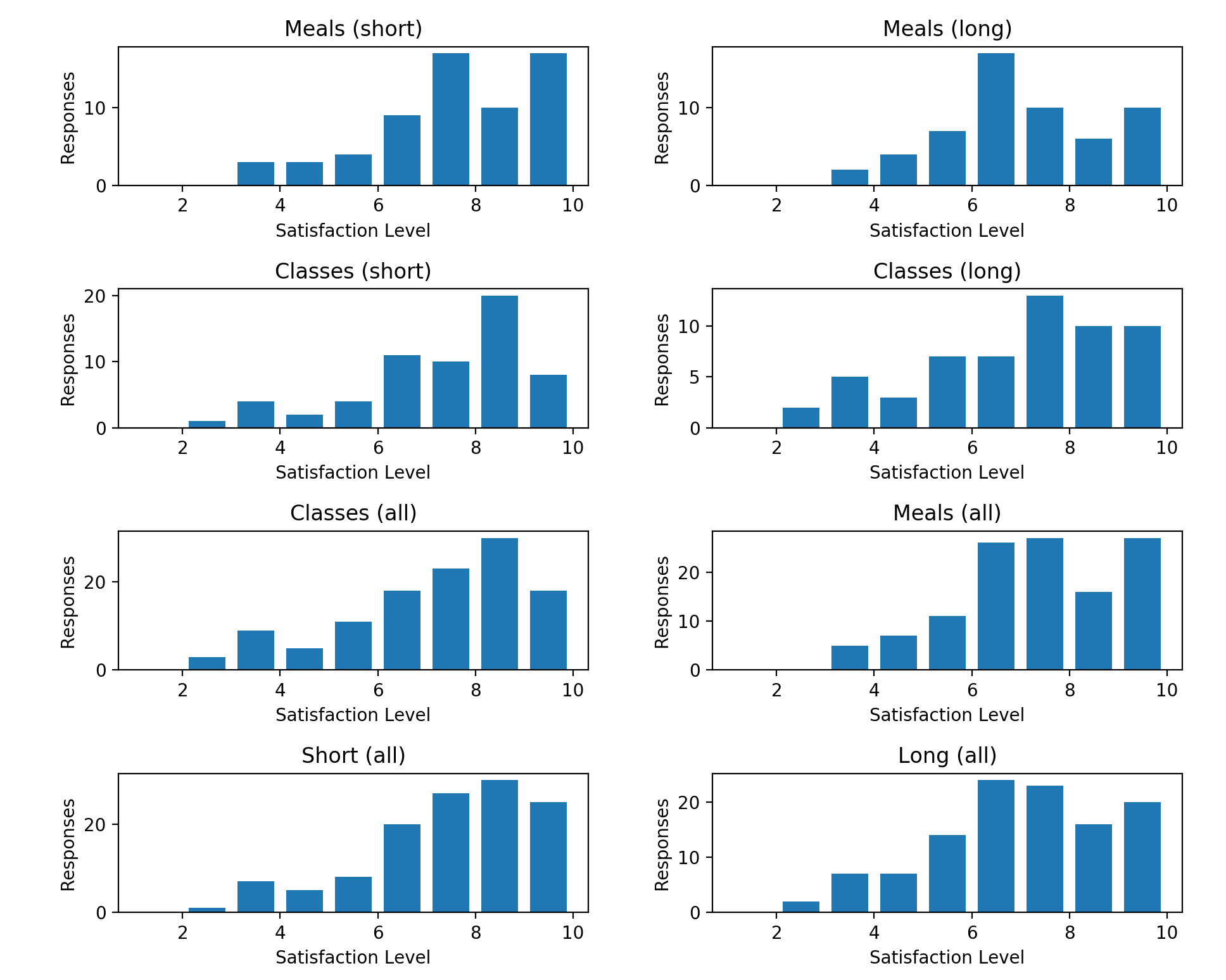{{ message }}

# vasilescur / DecSci-Group-9 Public

DECSCI 101 - Final Project (Group 9)

Switch branches/tags
Nothing to show

## Files

Failed to load latest commit information.
Type
Name
Commit time

# Decision Science 101 Final Project - Group 9

Analysis of the effect of multi/single-attribute decisions on the choice overload effect.

This repository contains the code used to analyze data collected from a Qualtrics survey.

## Data Processing

The data were exported from Qualtrics as a CSV format and then processed in Excel in order to remove irrelevant information such as IP address, date/time, and so on.

Then, the records were split into four different groups based on which columns were non-null:

```# Split into confidence values for each type of question
meal_short = data['meal_short'].dropna()
meal_long = data['meal_long'].dropna()

class_short = data['class_short'].dropna()
class_long = data['class_long'].dropna()```

## Statistical Analysis

### 2x2 ANOVA

The `statsmodels` package was used to perform a 2-way ANOVA. After the satisfaction values were aggregated into lists by category, the following code was used for the ANOVA:

```formula = 'Satisfaction ~ C(Type) + C(Length) + C(Type):C(Length)'
model = ols(formula, aggregate).fit()
aov_table = statsmodels.stats.anova.anova_lm(model, typ=1)```

The results of the ANOVA were:

``````                      df      sum_sq    mean_sq         F    PR(>F)
C(Type)              1.0    3.582745   3.582745  0.875582  0.350383
C(Length)            1.0   17.122664  17.122664  4.184584  0.041916
C(Type):C(Length)    1.0    0.300051   0.300051  0.073329  0.786790
Residual           233.0  953.399603   4.091844       NaN       NaN
``````

## Results Visualization

### Box & Whiskers Plot

Exploratory.io was used to create a data visualization. First, we used Excel to coalesce the data into the following format:

Type Length Response
class short 7
meal short 7
class short 6
class long 10
...

Then, the following box-and-whiskers plots were created from the newly formatted data:### Histogram Summary

In addition, the `pyplot` package from `matplotlib` was used to create a full visualization of the data consisting of a series of histograms outlining the preference reported by users for each category of question.

Graphs were creates as follows:

```plt.subplot(4, 2, 1)    # 1st plot
plt.title('Meals (short)')
plt.xlabel('Satisfaction Level')
plt.ylabel('Responses')
plt.hist(
meal_short,
bins=range(1, 11), # 1, 2, ..., 10
rwidth=0.75,
label='Meals (short)'
)

...

# Fix overlapping in layout, and then display the plots
plt.tight_layout()
plt.gcf().canvas.set_window_title('Results')    # Window title
plt.show()```

This resulted in the following visualization:## Website

This host is specified in the `CNAME` file in this repository, and the main HTML page template has been adjusted from the default Jekyll theme and can be found in `_layouts/default.html`.

## Project Contributors

• Zoe Tang
• Claire Hutchinson
• Prateek Khandelwal

DECSCI 101 - Final Project (Group 9)

## Releases

No releases published

## Packages 0

No packages published